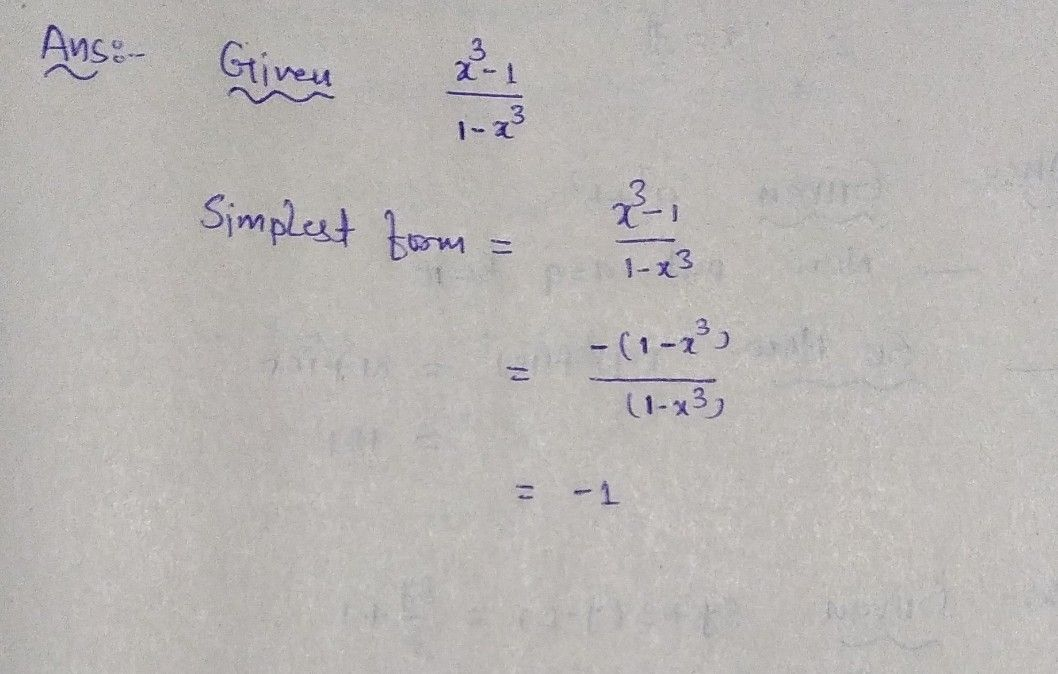Symbol
Problem9. Which of the following is the simplest form of $\dfrac {x-1} {1-x}7$ $A3$ $B$ $1$ $C-$ $-3$ $D$ $-1$
7th-9th grade
Other
Search count: 105
SolutionQanda teacher - kausharoption- D is correct